# getSimulationResults

Retrieve model simulation results and sample values used for computing Sobol indices

## Syntax

``[samplesTable,simdata,validRuns] = getSimulationResults(sobolObj,idx)``

## Description

example

````[samplesTable,simdata,validRuns] = getSimulationResults(sobolObj,idx)` returns the simulation results and sample values in the `SimBiology.gsa.Sobol` object `sobolObj` for the specified index `idx`. The index represents the ith column in `sobolObj.SimulationInfo.SimData`. This function is useful when you want to troubleshoot or find out which parameter samples generated the simulation data that resulted in failed model simulations.```

## Examples

collapse all

Load the Tumor Growth Model.

`sbioloadproject tumor_growth_vpop_sa.sbproj`

Get a variant with the estimated parameters and the dose to apply to the model.

```v = getvariant(m1); d = getdose(m1,'interval_dose');```

Get the active configset and set the tumor weight as the response.

```cs = getconfigset(m1); cs.RuntimeOptions.StatesToLog = 'tumor_weight';```

Simulate the model and plot the tumor growth profile.

`sbioplot(sbiosimulate(m1,cs,v,d));`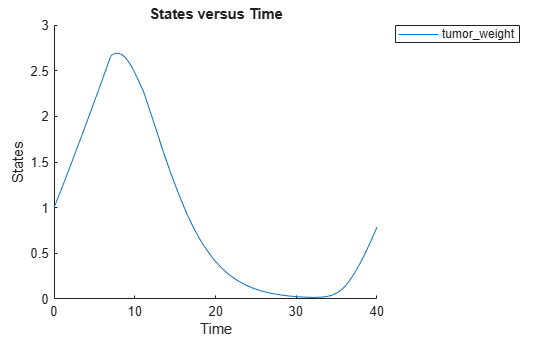Perform global sensitivity analysis (GSA) on the model to find the model parameters that the tumor growth is sensitive to.

First, retrieve model parameters of interest that are involved in the pharmacodynamics of the tumor growth. Define the model response as the tumor weight.

```modelParamNames = {'L0','L1','w0','k1','k2'}; outputName = 'tumor_weight';```

Then perform GSA by computing the first- and total-order Sobol indices using `sbiosobol`. Set `'ShowWaitBar'` to `true` to show the simulation progress. By default, the function uses 1000 parameter samples to compute the Sobol indices .

```rng('default'); sobolResults = sbiosobol(m1,modelParamNames,outputName,Variants=v,Doses=d,ShowWaitBar=true)```
```sobolResults = Sobol with properties: Time: [444x1 double] SobolIndices: [5x1 struct] Variance: [444x1 table] ParameterSamples: [1000x5 table] Observables: {'[Tumor Growth].tumor_weight'} SimulationInfo: [1x1 struct] ```

You can change the number of samples by specifying the `'NumberSamples'` name-value pair argument. The function requires a total of `(number of input parameters + 2) * NumberSamples` model simulations.

Show the mean model response, the simulation results, and a shaded region covering 90% of the simulation results.

`plotData(sobolResults,ShowMedian=true,ShowMean=false);`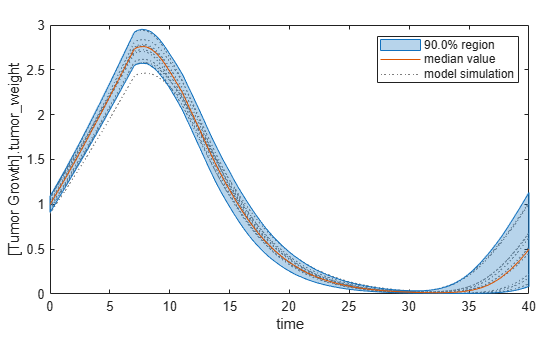You can adjust the quantile region to a different percentage by specifying `'Alphas' `for the lower and upper quantiles of all model responses. For instance, an alpha value of 0.1 plots a shaded region between the `100 * alpha` and `100 * (1 - alpha)` quantiles of all simulated model responses.

`plotData(sobolResults,Alphas=0.1,ShowMedian=true,ShowMean=false);`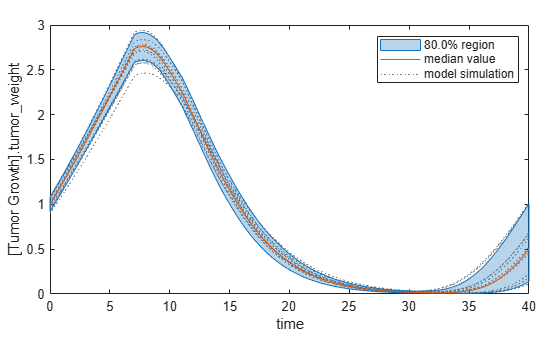Plot the time course of the first- and total-order Sobol indices.

```h = plot(sobolResults); % Resize the figure. h.Position(:) = [100 100 1280 800];```The first-order Sobol index of an input parameter gives the fraction of the overall response variance that can be attributed to variations in the input parameter alone. The total-order index gives the fraction of the overall response variance that can be attributed to any joint parameter variations that include variations of the input parameter.

From the Sobol indices plots, parameters `L1` and `w0` seem to be the most sensitive parameters to the tumor weight before the dose was applied at t = 7. But after the dose is applied, `k1` and `k2` become more sensitive parameters and contribute most to the after-dosing stage of the tumor weight. The total variance plot also shows a larger variance for the after-dose stage at t > 35 than for the before-dose stage of the tumor growth, indicating that `k1` and `k2` might be more important parameters to investigate further. The fraction of unexplained variance shows some variance at around t = 33, but the total variance plot shows little variance at t = 33, meaning the unexplained variance could be insignificant. The fraction of unexplained variance is calculated as 1 - (sum of all the first-order Sobol indices), and the total variance is calculated using `var(response)`, where `response` is the model response at every time point.

You can also display the magnitudes of the sensitivities in a bar plot. Darker colors mean that those values occur more often over the whole time course.

`bar(sobolResults);`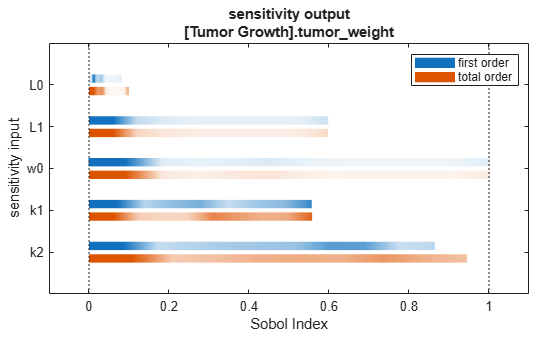You can specify more samples to increase the accuracy of the Sobol indices, but the simulation can take longer to finish. Use `addsamples` to add more samples. For example, if you specify 1500 samples, the function performs `1500 * (2 + number of input parameters)` simulations.

```gsaMoreSamples = addsamples(gsaResults,1500) ```

The SimulationInfo property of the result object contains various information for computing the Sobol indices. For instance, the model simulation data (SimData) for each simulation using a set of parameter samples is stored in the `SimData` field of the property. This field is an array of `SimData` objects.

`sobolResults.SimulationInfo.SimData`
``` SimBiology SimData Array : 1000-by-7 Index: Name: ModelName: DataCount: 1 - Tumor Growth Model 1 2 - Tumor Growth Model 1 3 - Tumor Growth Model 1 ... 7000 - Tumor Growth Model 1 ```

You can find out if any model simulation failed during the computation by checking the `ValidSample` field of `SimulationInfo`. In this example, the field shows no failed simulation runs.

`all(sobolResults.SimulationInfo.ValidSample)`
```ans = 1x7 logical array 1 1 1 1 1 1 1 ```

`SimulationInfo.ValidSample` is a table of logical values. It has the same size as `SimulationInfo.SimData`. If `ValidSample` indicates that any simulations failed, you can get more information about those simulation runs and the samples used for those runs by extracting information from the corresponding column of `SimulationInfo.SimDat`a. Suppose that the fourth column contains one or more failed simulation runs. Get the simulation data and sample values used for that simulation using `getSimulationResults`.

`[samplesUsed,sd,validruns] = getSimulationResults(sobolResults,4);`

You can add custom expressions as observables and compute Sobol indices for the added observables. For example, you can compute the Sobol indices for the maximum tumor weight by defining a custom expression as follows.

```% Suppress an information warning that is issued during simulation. warnSettings = warning('off', 'SimBiology:sbservices:SB_DIMANALYSISNOTDONE_MATLABFCN_UCON'); % Add the observable expression. sobolObs = addobservable(sobolResults,'Maximum tumor_weight','max(tumor_weight)','Units','gram');```

Plot the computed simulation results showing the 90% quantile region.

```h2 = plotData(sobolObs,ShowMedian=true,ShowMean=false); h2.Position(:) = [100 100 1280 800];```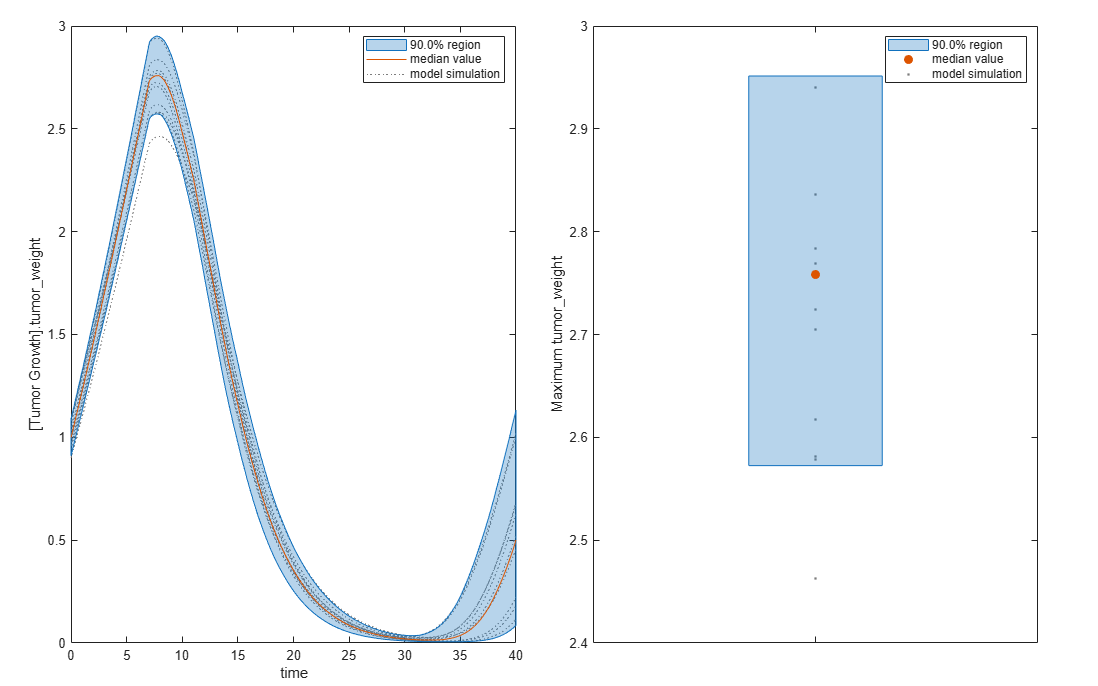You can also remove the observable by specifying its name.

`gsaNoObs = removeobservable(sobolObs,'Maximum tumor_weight');`

Restore the warning settings.

`warning(warnSettings);`

## Input Arguments

collapse all

Results containing the first- and total-order Sobol indices, specified as a `SimBiology.gsa.Sobol` object.

Index to extract the simulation results in `sobolObj.SimulationInfo.SimData`, specified as a positive integer. The index must be smaller than `params + 2`, where params is the number of input parameters. For more information, see Retrieve Simulation Results and Sample Values Using getSimulationResults.

Data Types: `double`

## Output Arguments

collapse all

Table containing parameter sample values, returned as a table.

Simulation results obtained using the samples in `samplesTable`, returned as a vector of `SimData` objects.

Indicators of run success or failure, returned as a logical vector. Each element indicates the success or failure of the corresponding simulation run in `simdata`.

collapse all

### Retrieve Simulation Results and Sample Values Using `getSimulationResults`

`getSimulationResults` returns the ith column from the SimData array `sobolObj.SimulationInfo.SimData`, which is one of the object properties.

As explained in Saltelli Method to Compute Sobol Indices, A and B are sample matrices. ${A}_{B}^{i}$ is a matrix where all columns are from A except the ith column, which is from B for i = 1, 2,…,params. params is the number of input parameters.

The simulation data used to compute Sobol indices is stored in `sobolObj.SimulationInfo.SimData`. The array size is `NumberSamples-by-params + 2`, where NumberSamples is the number of samples. The number of columns is `2 + params` because the first two columns correspond to simulation results from A and B. The rest of the columns correspond to ${A}_{B}^{1}$, ${A}_{B}^{2}$, …, ${A}_{B}^{params}$. In other words, you can consider the following:

`$\text{sobolObj}\text{.SimulationInfo}\text{.SimData}=\left[SimData\left(A\right),SimData\left(B\right),SimData\left({A}_{B}^{1}\right),SimData\left({A}_{B}^{2}\right),...,SimData\left({A}_{B}^{params}\right)\right]$`

Here, the first column corresponds to simulation data using the sample matrix A, the second column corresponds to simulation data using the sample matrix B, the third column corresponds to simulation data using the matrix ${A}_{B}^{1}$, and so on.

For instance, `getSimulationResults(sobolObj,3)` returns:

• `samplesTable` (first output), which is ${A}_{B}^{1}$.

• `simdata` (second output), which contains simulation results using the samples from ${A}_{B}^{1}$. This is the third column of `sobolObj.SimulationInfo.SimData`.

• `validRuns` (third output), which contains logical values that indicate the success or failure of each simulation run in `simdata` (second output). `validRuns` corresponds to the ith column of `sobolObj.SimulationInfo.ValidSample`.

 Saltelli, Andrea, Paola Annoni, Ivano Azzini, Francesca Campolongo, Marco Ratto, and Stefano Tarantola. “Variance Based Sensitivity Analysis of Model Output. Design and Estimator for the Total Sensitivity Index.” Computer Physics Communications 181, no. 2 (February 2010): 259–70. https://doi.org/10.1016/j.cpc.2009.09.018.